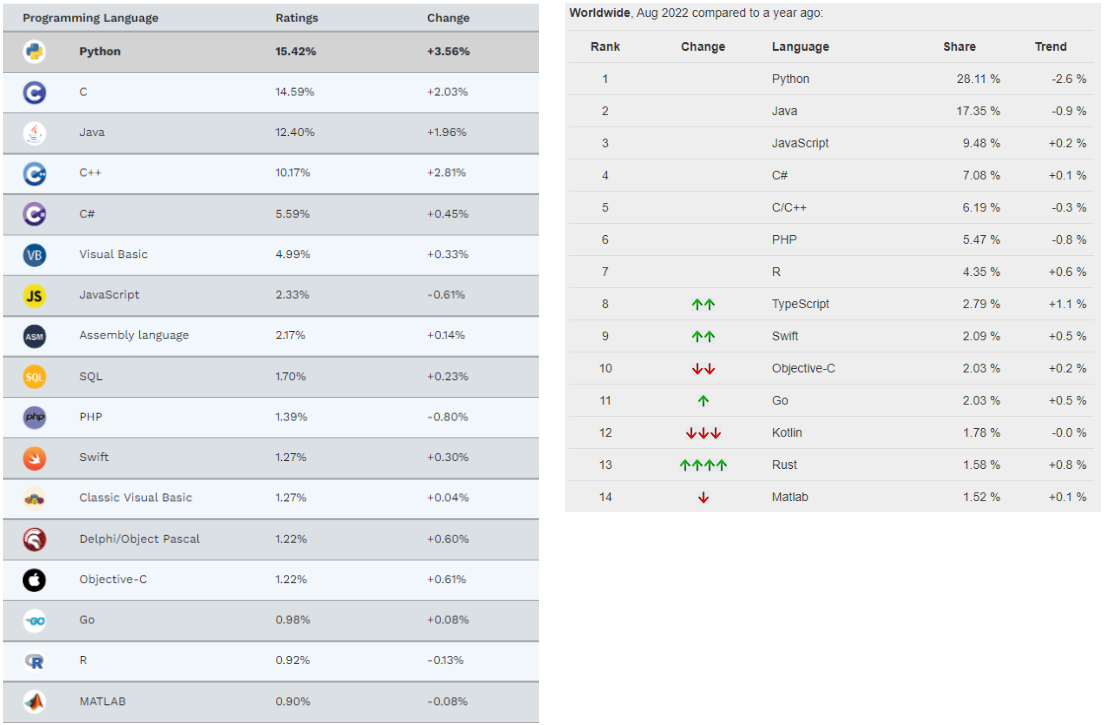May 21, 2022

# Comparing Matlab vs Python for Systems Design and EngineeringWe find ourselves in a world teeming with data. Many companies work hard to use data to ensure they are among the first in recognizing and adapting to market shifts. Python and Matlab are the main programming languages used to analyze and process vast amounts of data. This is especially true in engineering, scientific and computational domains.

Numerous Python vs Matlab debates have been had regarding which programming language is better. We will attempt to summarize the key differences and introduce you to Collimator which is built to shore up Python's major weakness (see excerpt below) and hopefully signal a turning point in the debate.

## Why is Python so popular?Ranking of most popular programming languages - TIOBE (L) and PYPL (R)

According to the TIOBE and PYPL Popularity of Programming Indices, Python is the top programming language today. This is likely because it is a free, open-source, easy to learn, high level general purpose programming language. Python presents itself as a solution to the vast majority of the world's data needs and boasts several key features including:

1. Easy Syntax - Python's syntax is similar to English. With just a few hours of learning Python, a data scientist or engineer can easily create a neural network. Below is a simple example with PyTorch

class Net(nn.Module):
def __init__(self):
super(Net, self).__init__()

# First 2D convolutional layer, taking in 1 input channel (image),
# outputting 32 convolutional features, with a square kernel size of 3
self.conv1 = nn.Conv2d(1, 32, 3, 1)
# Second 2D convolutional layer, taking in the 32 input layers,
# outputting 64 convolutional features, with a square kernel size of 3
self.conv2 = nn.Conv2d(32, 64, 3, 1)

# Designed to ensure that adjacent pixels are either all 0s or all active
# with an input probability
self.dropout1 = nn.Dropout2d(0.25)
self.dropout2 = nn.Dropout2d(0.5)

# First fully connected layer
self.fc1 = nn.Linear(9216, 128)
# Second fully connected layer that outputs our 10 labels
self.fc2 = nn.Linear(128, 10)

my_nn = Net()
print(my_nn)



2. Efficiency - Python is efficient; less code produces more work. Today, it is not uncommon for programmers to write solutions in Python and then convert the code into other languages. Below is an example where Python uses half the code to produce the same output when compared to Java

3. Robust External Libraries - Python has over 100,000 open source standard libraries that engineers can use while developing their own systems. These Python libraries range from data processing libraries including SciPy, NumPy and Matplotlib to domain specific libraries. Examples of these include AI libraries such as Google's TensorFlow and Facebook’s PyTorch

4. Diversity - Python is as diverse as the community that engages with it. Data science teams exist in almost every industry, function and company. These teams heavily rely on Python to conduct data analysis using statistics or even AI and machine learning

## Python vs MATLAB trends

MATLAB is platform independent and its roots lie in numerical computing. Like Python it is intuitive and easy to use, and its Simulink toolbox provides a rich visual platform to manipulate data graphically and model and visualize block diagrams easily. However, unlike Python, MATLAB is proprietary and expensive leading to some analysts suggesting that MATLAB is waning in popularity.

Some of the reasons why include:

1. Narrow Scope - MATLAB started out as a tool for scientific computing, therefore it seems closer to a scripting language than a high level general-purpose language. Python, on the other hand, provides all the mathematical power that MATLAB boasts, but in the context of a general purpose programming language.

2. Proprietary - MATLAB is a proprietary language which limits the ability for people to build on top of it. Python is open source and it's functionality has been extended through useful open source libraries such as SciPy, NumPy and Matplotlib. This makes coding in Python much more efficient

3. Slow Speed - MATLAB code shares the same interpretive structure as Python. Code is run line by line by an interpreter instead of being compiled all at once. As a result, both MATLAB and Python are much slower than compiled languages like C++ and Java. Python justifies the significant speed deficit it suffers by being extremely productive and versatile. Paul Romer, the 2018 Nobel Prize in Economics, put it best when he wrote, “9/10 times Python’s slow performance doesn’t matter.

## Introducing Collimator, a “Simulink for Python”

One area that Python has remained absent has been in GUI simulation and model-based development. There has been no Python based solution that has been able to provide the richness that Simulink provides. In the wake of the explosion of open-source software, it’s not surprising that companies have launched tools like Scilab and Xcos, to solve this pain point. In spite of this, none of these tools have reached mass adoption.

At Collimator, our goal is to build engineering software that can be used for model-based design and allow engineers to code in the way that they are most comfortable. Therefore, Collimator’s development environment seamlessly combines Python with a block diagram, graphical user interface on the cloud.